Courses

# Test: Fluid Flow Kinematics - 3

## 10 Questions MCQ Test Topicwise Question Bank for Civil Engineering | Test: Fluid Flow Kinematics - 3

Description
This mock test of Test: Fluid Flow Kinematics - 3 for Civil Engineering (CE) helps you for every Civil Engineering (CE) entrance exam. This contains 10 Multiple Choice Questions for Civil Engineering (CE) Test: Fluid Flow Kinematics - 3 (mcq) to study with solutions a complete question bank. The solved questions answers in this Test: Fluid Flow Kinematics - 3 quiz give you a good mix of easy questions and tough questions. Civil Engineering (CE) students definitely take this Test: Fluid Flow Kinematics - 3 exercise for a better result in the exam. You can find other Test: Fluid Flow Kinematics - 3 extra questions, long questions & short questions for Civil Engineering (CE) on EduRev as well by searching above.
QUESTION: 1

Solution:
QUESTION: 2

Solution:
QUESTION: 3

### The basic principles of fluid flow are 1. conservation of mass 2. conservation of energy 3. conservation of momentum Which of these statements are correct?

Solution:
QUESTION: 4

The mean velocities at two ends of a stream tube 10 cm apart are 2.5 m/s and 3 m/s. The convectional tangential acceleration mid-way is

Solution:

Convectional tangential acceleration is given by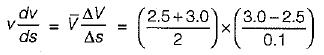= 13.75 m/sec2

QUESTION: 5

The flow of water in a wash hand basin when it is being emptied through a central opening, is an example of

Solution:

Free vortex flow is irrotational flow and total head (energy) remains constant throughout flow field.

QUESTION: 6

Given the x - component of the velocity u = 6xy - 2x2, the y-component of the flow velocity is given by:

Solution:

The flow must satisfy continuity equation,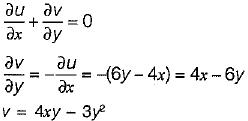QUESTION: 7

Which one of the following represents two dimensional rotational flow?

Solution:

For rotational flow,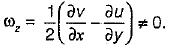So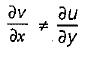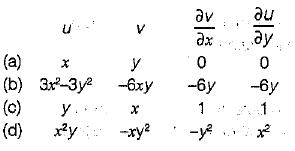QUESTION: 8

If flow velocity in x and y directions are given by u = 2x + 3y and v = - 2y. The circulation around the circle of radius 2 unit will be

Solution:

u = 2x + 3y
v = - 2y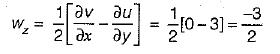vorticity = 2 x rotational velocity
= - 3
circulation = vorticity x area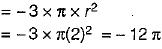QUESTION: 9

Match List-I (stream line pattern) with List-II (type of acceleration) and select the correct answer using the code given below the lists: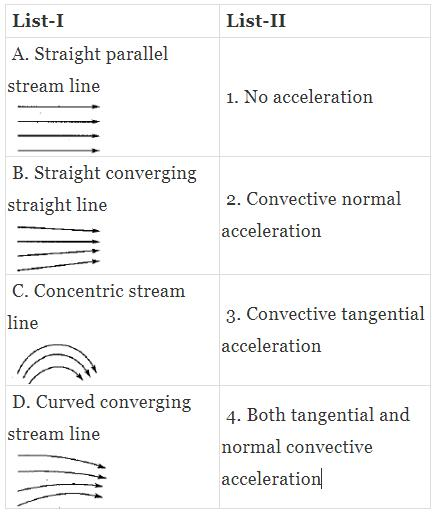Codes: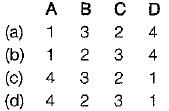Solution:
QUESTION: 10

A stream function is given by, ψ = 2x2y + (x + 1 )y2. The flow rate across a line joining points A(3, 0) and B(0, 2) is

Solution: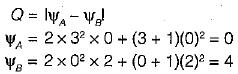∴ Q = |0 - 4I = 4 units.

Track your progress, build streaks, highlight & save important lessons and more!

### Similar Content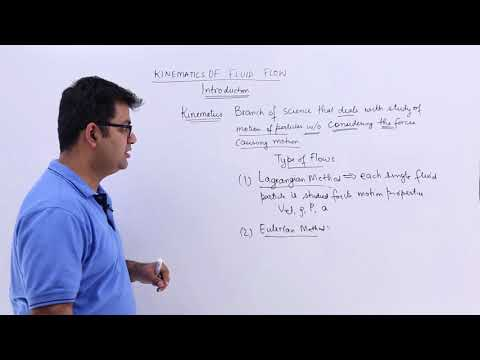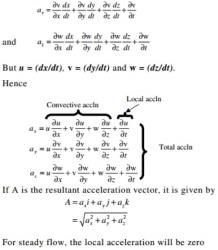### Related tests